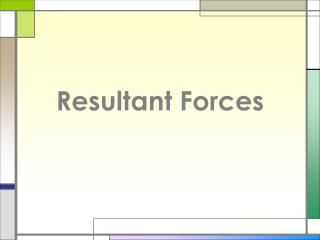DownloadDownload PresentationResultant Forces

# Resultant Forces

Télécharger la présentation## Resultant Forces

- - - - - - - - - - - - - - - - - - - - - - - - - - - E N D - - - - - - - - - - - - - - - - - - - - - - - - - - -
##### Presentation Transcript

1. Resultant Forces

2. If two forces act together on an object, their effect may be described as the action of one force.

3. EXAMPLE Two forces of magnitude 5N and 6N act on a particle. They act at right angles. Find the magnitude and direction of their resultant.

4. EXAMPLE Two forces acting on a particle have magnitudes 8N and 3N. The angle between their directions is 60°. Find the resultant force acting on the particle.

5. EXAMPLE Three forces (2i – j) N, 3i N and (-i + 4j) N, where i and j are unit vectors due east and due north respectively, act on a particle. Find the magnitude and direction of the resulting force.

6. EXAMPLE Forces P, Q and R act on a particle at O in the plane of the coordinate axes Ox, Oy, making angles p, q, r respectively with Ox in the first quadrant. P = 4 N, Q = 3 N, R = 6 N, p = 30°, q = 45°, r = 60°